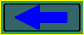﻿ Logic and experimental addition to a set of equations of Maxwell.

Addition to Maxwell's equation &   Ampere's Law.
Logic, calculations, experiment.

The insufficiency of the theorem about circulation of a vector of a magnetic intensity in the electric circuits, which include the closed and hollow conductor, is shown.

It is impossible to create separate, unenclosed part of a conductor, with flow of the direct current (the separate, unenclosed element of a DC) - it disturbs a conservation law of a charge (Fig. 1).Fig. 1

Therefore it seems obvious, that it is impossible to create a magnetostatic field (MF) by means of a separate part of a direct current [1, page 163]. The prime logic of this conclusion - there is no object for reviewing. Thus, the concepts "the separate, unenclosed element of DC" and "a separate element of a DC" - the part of closed electric circuit, are equated. On the basis of this logic the known experimental facts are interpreted and the conclusions in the theory of an electromagnetism are made.

Let's test completeness of this logic.

Let's consider only electric circuits of a direct current.

Nomenclature:

1) A conductor - the body, in which the charge particles are moving (electrical current), if inside a conductor the electric field strength E is distinct from zero. [1, page 26].

2) The inverse square law.
It is the law on central fields created by centres of force (gravitation masses, electrical charges, magnetic poles) under the law of inverse proportionality to square of distance. It is spoken about identical description of such fields [1, page 19], [1, page 46]. It is known, inside of an gravitating hollow orb the some body does not feel the gravitational force.

3) In space around an arbitrary current, always exists a MF [1, page 161].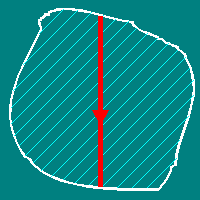Line conductor and area of it�s MF

4) The closed conductor - the body which ensures course of a current in the closed linear circuit [1, page 164, 167]. Usually this is a single-path circuit. Ones make the circuit of a linear, metal conductor. The current flowing in a closed conductor, creates MF in all space around itself (see item 3).

5) Biot-Savart relation in the vector form [1, page 163] :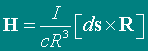I - current intensity in a conductor; ds - element of a linear conductor; R - Radiuses-vector from current element Ids, which create a MF, up to a point, where strength of H of it MF is observed.

6) The closed and hollow conductor (CHC) - conductive body which is made as a continuous closed surface completely enveloping some volume [2, page 65]. Usually it is a surface of rotation - cylindrical or spherical. Cross-points of a surface and symmetry axis - poles. The current, which flows on a surface of a CHC between poles, does not create a MF in volume, which it circulates. The same but more precisely - the vector sum of magnetic fields which are created by all elements of a current, in any point inside of a CHC is equal to a zero.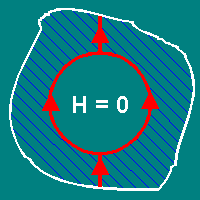Closed and hollow conductor and area of it�s MF

This property of CHC follows from Biot-Savart relations and of "inverse quadrates" (see item 2, item 5). Otherwise it is necessary to assume, that the intensity of MF, created by a current element, varies under the law distinct from law of inverse proportionality to quadrate of distance, but it contradicts experience. The same property of CHC confirms by numerical calculations.
So, in the closed electric circuit there can be conductors with current of two types:
1) Which create MF everywhere; 2) Which create MF everywhere excluding some volume which they cover.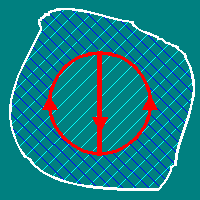Closed circuit of two types of conductors and area of it�s MF

A minimum number of both types of conductors which are necessary for making a closed electric circuit - two (Fig. 2, Fig. 3).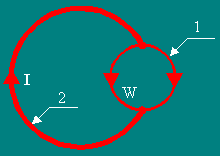Fig. 2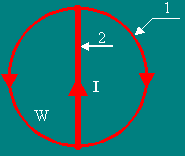Fig. 3

On figures are designated: 1 - spherical CHC (section); 2- a linear conductors; I - a current flowing in a circuit; W - a volume enveloped of CHC.

Let's analyse a circuit shown in a Fig. 2.

Let's designate of a pole of a CHC as A and B (Fig. 4).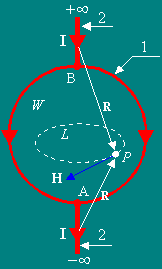Fig. 4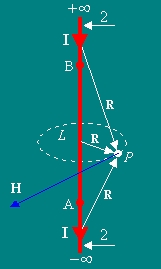Fig. 4a

A linear conductor 2, we shall present as semi-infinite rectilinear parts -A and +B. They are located on one axis. The created circuit with a current has a rotational symmetry. Here a MF force lines have an azimuthal (tangential) component only. They are circles with centre on an axes of a system. Let's consider a point P. It belongs to a contour L. The contour L is in a volume a W and coincides with a guessed force line. The CHC envelops a volume W.

Let's discover circulation of a vector of a magnetic intensity on a contour L.
Definition: �If the curve L is closed (it is marked by a circle on the sign of an integral), the line integral of a vector a along L is termed as circulation of a vector a lengthways L [1, page 469]�: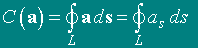(23*)

Now we'll designate: an element of a contour L as dl and element of a circuit Ls as ds.

Because in our case the vector H is always parallel to dl, so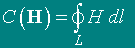According to a principle of superposition for a circuit with a current: �...the field strength H of the closed current I in an arbitrary point P is equal to the sum of fields created by each of its elements, i.e. is equal: [1, page 164] � :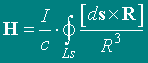(42.4)

In this expression it is meant, that the current flows in a linear closed circuit, on which the integration is made.

In case of an infinite rectilinear conductor conterminous with an axis of viewed system (Fig. 4a), all its elements would give the contribution in a MF in a point P (R - Radiuses-vectors from current elements to a point of observation P ).

However, in a considered case, the current, which flows on CHC 1, does not create MF in a volume W (item 6) and the formula (42.4) will be so: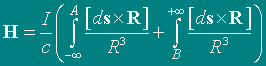and circulation of a vector of a magnetic intensity on a closed curve L will be :It is obvious, in a considered circuit the circulation of a vector of a magnetic intensity on a closed contour L (which not envelop of currents) is not equal to a zero, and the guessed force line became real.
It contradicts the theorem of circulation of a vector of magnetic intensity, in which is spoken that: "...the circulation of a vector of a magnetic intensity on a curve which not envelop of currents, is equal to a zero... " [1, page 178].

Whence this conflict? Let's try to understand.

The Stokes theorem..

The circulation of an arbitrary vector a on a closed curve L is equal to a stream of a curl of this vector through a surface S which based on a curve L � [1, page 472]: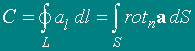It was deduced the formula which without discussion is applied for a long time [1, page 177]:(47.3)

The theorem of the Stokes is only mathematical. There is no word in this theorem about a stream of charges through a surface S. However, if to view physical application of the theorem, the curl and stream of a curl is created by this stream of charges.
Nevertheless, ones says: " On the basis of the theorem of the Stokes and equation (47.3) we can write [1. page 177] � :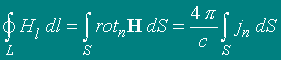(47.4)

It is obvious, that there is a direct substitution of a curl of a vector by a current vector, which forms this curl. It is consequence of that "obvious" logic of reasoning. It is possible to make a conclusion, that the equation (47.3) in this case is untruly and requires theoretical finishing, and "...a complete set of the differential equations of a magnetic field of direct currents..." [1. page 182] is not complete, as well as set of Maxwell's equations - NOT OBVIOUSLY AND NOT NECESSARILY OBSERVANCE OF EQUALITY:It has taken place because an electric current, including DC, is the ordered (organized) motion of charge carriers. Ordered driving charge carriers of the definite sign generate a magnetic field.
The magnetostatic field is a random, statistical superposition of elementary and driving charge carriers which create a constant electric current.
Therefore, considering any system of direct currents, with reference to a magnetic field, created by them, it is necessary to apply a principle of superposition and Biot-Savart relation to each driving charge carrier - source of a variable electrical field .
Then in an equation (47.3), which full form looks as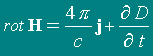for consideration there is, only, a second term of its right member.

Ones more. It has taken place because in times, when the Ampere law was stated, there was no opportunity of numerical calculation of a magnetic field inside CHC, i.e. field created by all the driving charge carriers on a surface of CHC and between its poles.
The analytical expression of such vector field contains an elliptic integral of the second sort which can not be transformed to elementary functions for simple numerical calculations. The theorists explored only linear contours with a current. This care was quite justified. Gradually it became a norm and the theorem of circulation of a vector of intensity of MF became a scientific dogma. Because of it other systems with a current further were not counted. It has braked development of the theory and based on it an applied knowledges.

Briefly we shall iterate logic of a conclusion.

1) Or there are two types of conductors with direct current ( 1 - which create a MF in all space; 2 - which create a MF in all space excluding a part of space, which they envelop) - or a Biot-Savart relation and law of "inverse quadrates" are untrue.

2) Or there are a closed electric circuits of a direct current, where the circulation of a vector of a magnetic intensity on a closed curve L which not envelop a currents, is not equal to a zero - or the principle of superposition is untrue.

Let's consider consequences of these reasoning.

At first, it is necessary to eliminate perpetuity out the formulas and to create a real physical system.

Let's create a rotationally symmetric closed circuit (Fig. 5) consisting of two linear conductors AC, BD and two spherical CHC 1 and 2 which are inserted one in another and not touch each other. The linear conductor BD connect the upper poles of CHC 1 and 2. The linear conductor AC connect the low poles of CHC 1 and 2. The arrows show a direction of currents in a circuit.
On a Fig. 5a the possible variant of connecting of power supply to circuit is shown.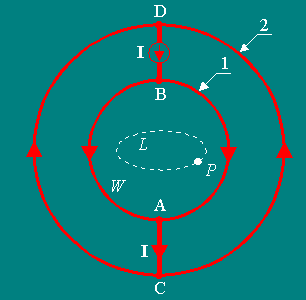Fig. 5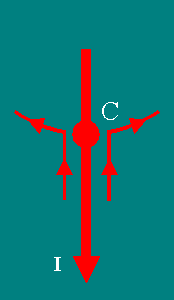Fig. 5a

Now formula (42.4) will be so:and the circulation of a vector of magnetic intensity on a contour L will be: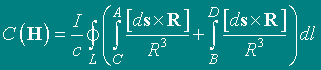because CHC 1 and 2 does not create a MF in a volume a W(item 6). It is obvious, that a MF in a volume a W is a sum of a MF of separate parts of a circuit - AC and BD.

Calculated structure of a magnetic field of such device in a plane containing a symmetry axis is shown on Fig. 6 as the graph of the Cantor.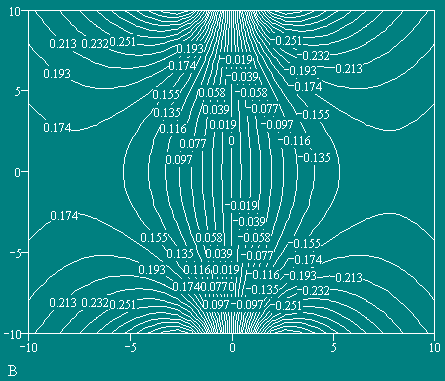The surfaces of a level of equal magnetic intensity are shown.
The direction of vector of magnetic intensity is perpendicularly planes of page.

Fig. 6

Such a MF has the following singularities :
1) Line of a zero field strength (LZFS);
2) Minimum of magnetic intensity by on three coordinates at centre of a system (excluding LZFS);
3) The gradient such, that a field strength increases on a direction from centre of curvature of force lines.

Thus, for a research and application the new class of magnetostatic fields - a fields created by separate cuts of a direct current is entered.

In a nature such a MF exists in any channel with current, where the current bypasses some hindrance. In such channel with a current I (Fig. 7) always is possible to find a tube of a current i (down to separate mobile charged corpuscles - MCC), which diameter d is less than a size D of hindrance. So can be formed ball-lightning in the channel of a linear lightning .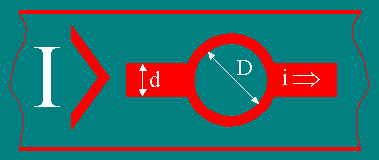Fig. 7

Such MF exists between two MCC, moving in one line to one side (Fig. 8).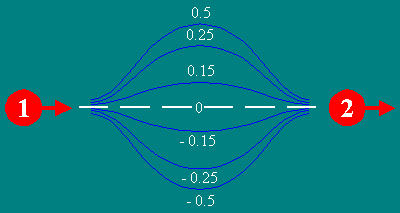Fig. 8
Some of the surfaces of a level of equal magnetic intensity are shown.
The direction of vector of magnetic intensity is precisely perpendicularly planes of page
.

The configuration of MF (Fig. 9) is similar to a field in "mirror machine" , but with the "reflecting relation" more than 100 and with force lines, which have an azimuthal (tangential) component only. Because of an explicit likeness, such MF was named a "magnetic cocoon". The configuration of MF in "a magnetic cocoon" corresponds to a requirement of a minimum of a field strength . It allows to create for a long time expected trap for plasma and industrial controllable thermonuclear reactor with a magnetic plasma confinement . Such MF is found experimentally at toroidal current structures (see further).Fig. 9
Some of force lines of one of surfaces of equal potential are shown.
The sections of those surfaces are represented in a Fig. 8
.

The research of behavior of free radicals in variable MF of such configuration is of interest. Formation of spiral polymeric (organic) structures here is possible.

According to calculations, a MCC of the plasma in a "magnetic cocoon" will create toroidal formation with a poloidal vector of a corpuscles velocity and R r - caustic (Fig. 10).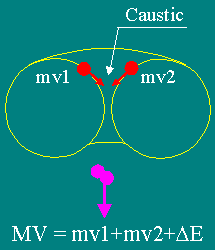Fig. 10

At increase a MF, the Larmor radius of MCC will diminish - the plasma will be pinched comprehensively. The effect is named a "spherical pinch�. It is possible to create toroidal formation where the density of charges in a toroidal stratum will be much higher, than in metals. The refraction coefficient of a gamma-rays will rise - probably making of effective gamma-rays optics.

In a caustic there will be collisions of corpuscles and to flow reactions of kernel synthesis of hydrogen cycle, carbon cycle and other cycles. The toroidal formation can change a size under influence of exterior forces. It will change MF in a system - it is possible to create the detector of these forces.

If MCC fly under an angle in MF of such configuration, they will be reflected from of "magnetic walls" (Fig. 11).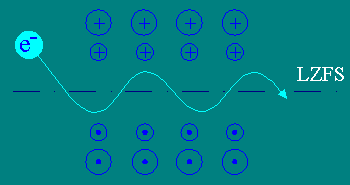Fig. 11

It is equivalent to a system of MF in undulator. It is systems which use in a relativistic electronics engineering for making a free electron laser [6, page 486].

Now on a basis a MF of such configuration are develop the devices :

THE UNIVERSAL MAGNETIC LENS belongs to electronic optic technology. Can be used as dispersing lens. Also may be used for a heightening of a resolution capability of electron-optical systems at 5-10 times at the expense of elimination of aberrations.

THE REGULATED MAGNETIC UNDULATOR belongs to area of accelerators technology. The period of a undulator can smoothly be regulated (from a share of millimetre up to centimetres). It can be utilised for making free electron lasers and as an controllable radiantes of electromagnetic oscillations in wide (UHF - GAMMA) a frequency band and an output power.

THE DEVICE FOR A PLASMA CONFINEMENT belongs to area of plasma technology. It can be utilised for making plasma devices, for jet drives of new generation and for independent, ecological pure, safe and controllable sources of a power from 0.01 to 10 MW with a specific power 10 - 20 kW / kg.

The device shown on a Fig.5 can be easily transformed into two axial toruses (Fig. 12). The properties of toroidal current structures will be surveyed later.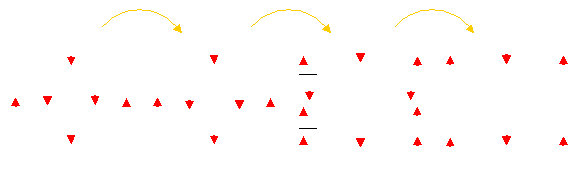Fig. 12

Now we shall consider an electric circuit shown on a Fig. 3.

The theorem of circulation of a vector of a magnetic intensity says: �...circulation of a vector of a magnetic intensity on a curve... enveloping a currents, is equal multiplied on 4p/c the sum of forces of these currents (taken with suitable signs)� [1, page 178]. From the theorem follows, that the circulation is constant and does not depend on geometry of a circuit.

Let's test the theorem.

Let's create a rotationally symmetric closed circuit (Fig. 13). It consist of a spherical closed and hollow conductor (CHC) 1 and linear conductor 2. The linear conductor connect a poles of CHC. The arrows show a direction of currents in a circuit.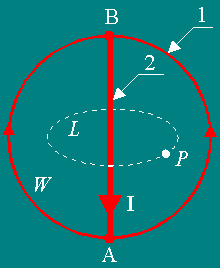Fig. 13

Because of a symmetry the force lines of MF have an azimuthal (tangential) component only. They are circles with center on an axes of a system. Let's consider a point P. A point P belongs to a contour L. The contour L coincides with a force line.
Let's discover circulation of a vector of strength of MF on a contour L. The circulation of a vector H along a closed curve L is defined by expression :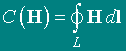In our case H is parallel dl andTo be convinced of validity of the theorem, it is necessary and enough to prove, that the strength of MF in a point P is constant at a changing of sizes of a system (a contour of an integration L and intensity of current I in a linear conductor are constants). CHC 1 does not create MF in volume which it envelops (item 6). Therefore, according to a principle of superposition, it is possible to consider only a linear conductor AB (Fig. 14).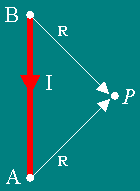Fig. 14

By the same principle, the strength H of MF of a current I in an arbitrary point P is equal to the sum of fields of its elements :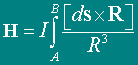I - intensity of current in a conductor; ds - element of a circuit; Ids - current element;
R - Radiuses-vector from current elements to a point of observation P.

Let's increase sizes of a system (Fig. 15).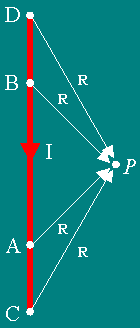Fig. 15

CHC 1 still does not create a MF in volume, which it envelops (item 6). The intensity of MF in a point P because of the extra contributions of pieces of a current AC and BD will be increase and becomes :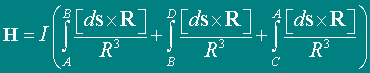It is obvious that the intensity MF in a point P changes at change of the sizes of system. That contradicts a position of the theorem and that was necessary to show.

The electric circuit shown on Fig. 3 also can be transformed into a torus.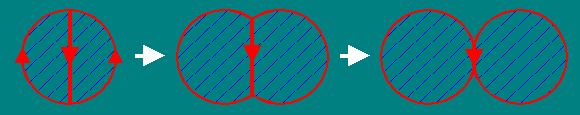Transformation a closed circuit to torus and area of it�s MF
(The classical representation, where all a MF is focused inside a torus).

Equivalence of MF, created by a current on linear and on hollow cylindrical conductors.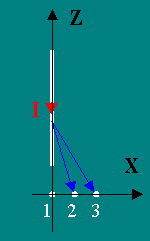Fig. 16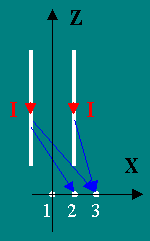Fig. 17

It is obvious, that the magnetic intensity in point 1 will be º 0. After step by step approaching a point 2, H will be to increase, and after passage of a maximum (the point 3 whose placement depends on geometry of a circuit ), will to decrease under the law which is distinct from 1/R or 1/R2.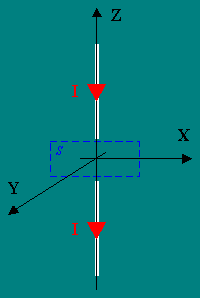Fig. 18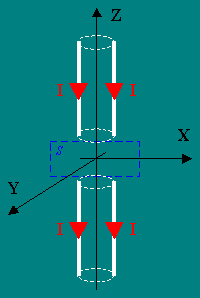Fig. 19

Now, on the basis of the shown equivalence, the transformation of a circuit (Fig. 3) can be finished up to full torus. Here length of a cylindrical conductor aspires to zero.
The calculation of MF of torus will be shown further.List of the literature.

1) "Tamm, Igor Yevgenyevich" Encyclop?dia Britannica Online. I. E. Tamm // "Fundamentals of the theory of an electricity", Moscou, Science, Main edition of the physical and mathematical literature, 1989.

2) S. G. Kalashnikov // "A Common Course of Physics", vol. 2, state publishing house of the engineering and theoretical literature, Moscou, 1956.

3) S. Yu. Taskaev // Plasma physics, Sept., 1997, vol. 23, ?12, p.1123; "Dynamics of the Potential of a Plasma Jet Heated by Atomic Beams in a Mirror Machine".

4) T. S. Simonen // Plasma physics, Sept., 1997, vol. 23, ?9, p.771; "High Pressure Plasma Stability with Favourable Magnetic Field Lines Curvature".

5) S. V. Putvinskij// UFN, Nov. 1998, vol. 168, ?11, p.1235; "Can the future world energy system be free of nuclear fusion".

6) "The Physical encyclopaedic dictionary", chapter the editor A. M. Prokhorov, Moscou, " The Soviet Encyclopedia ", 1983.